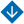No. 3, 2017
•About Advisory & Editorial Boards Aims & Scope Publication Ethics Review Process Archive Contact Information

#No. 3, 2017

Surveys

Shvedov A.S. Fuzzy Mathematical Programming: A Brief Review (pp. 2-10)

Abstract. The survey is given of several fuzzy mathematical programming chapters. It is observed that related to fuzzy mathematical programming are the mathematical programming tasks being formulated with the use of the fuzzy set theory concepts in any way. Considered are the tasks with soft constraints, tasks with fuzzy parameters, ranking functions, possibility measures. Fuzzy random mathematical programming tasks are discussed on the example of securities portfolio selection problem.

Keywords: fuzzy mathematical programming, possibility measures, fuzzy random variables.

Analysis and Design of Control Systems

Zheleznov K.O., Khlebnikov M.V. Feedback Design for Linear Control System with Disturbance in Both Input and Output: Robust Statement (pp. 11-16)

Abstract. The design problem for linear control system with uncertainties in the system coefficients and exogenous disturbances is considered. The solution technique is based on the invariant ellipsoids approach. The efficacy of the proposed method is demonstrated via the benchmark problem for the aircraft model.

Keywords: linear control system, linear matrix inequalities, invariant ellipsoids, bounding ellipsoids, robustness.

Chestnov V.N., Samshorin N.I. Controllers Design via Given Oscillation Index: Parametric Uncertainty and Power-Bounded External Disturbances  (pp. 17-25)

Abstract. The problem is considered of output controllers design for linear multivariable systems with deviating in prescribed bounds physical parameters of the plant, and subjected to the influence of unknown polyharmonic external disturbances, limited only in power. The controller is built so that to provide the prescribed bounds of mean-square values of controlled variables in addition to the robust stability of the closed-loop system. The problem solution is reduced to the H-optimization procedure produced in some specific way.The solution of the well-known «benchmark» problem is considered.

Keywords: robust stability, oscillation index, external disturbances bounded in power, Н-control.

Control in Social and Economic Systems

Murinovich A.A., Loginov M.P. Management Framework of Inter-Regional Projects and Programmes Portfolio in Russia (pp. 26-36)

Abstract. The necessity is considered of portfolio approach application for inter-regional and regional projects and programmes management in the federal district territory. The peculiarities are given of inter-regional projects portfolio management, the specific features are spotted of projects’ selection, realization process planning, and the portfolio operational management.

Keywords: portfolio, inter-regional project, projects and programmes portfolio management, project selection, planning.

Information Technologies in Control

Tolok A.V., Tolok N.B. Mathematical Programming Problems Solving by Functional Voxel Method (pp. 37-42)

Abstract. One of the approaches to mathematical programming problems solving by means of R-functional modeling is considered. Some functional voxel method tools are presented for the wide class of optimization problems computer solution using voxel algorithm of gradient descent. The illustrative examples of the computer calculations in comparison to non-computer solutions are given.

Keywords: R-function, R-functional modeling, voxel, local geometrical characteristics, the functional voxel method, functional voxel model, M-image, mathematical programming.

Podlazov V.S. Internal Parallelism of System-Area Networks with Incremental Diameter (pp. 43-48)

Abstract. The problem is considered of increasing the parallelism of system-area networks by adaptive routing at the minimal network diameter extension. The problem is solved experimentally for the complete multiring and the generalized hypercube. The empirical formulas of the minimum number of different routes are obtained for the networks with nodes number up to hundreds thousands. The system-area networks are proposed to be used in incremental diameter mode as a means of increasing their processing rate and channel fault-tolerance.

Keywords: system-area supercomputer networks, complete multiring, generalized hypercube, flattened butterfly, parallel of network, network diameter, network throughput, channel fault-tolerance.

Control in Medical and Biological Systems

Babushkina N.A., Glumov V.M., Kuzina E.A.

Abstract. On the basis of the antitumor vaccine therapy mathematical model the algorithm is developed for calculating the dosage and viral vaccine introduction moment, at which the immune system response to the virus and infected tumor cells is the most effective. The method is developed of calculating the experimental tumors maximum allowable size, the excess of which reduces the efficiency of the vaccine therapy. The method is based on the mathematical description of the dynamics of fast-proliferating tumor cells fraction decrease with the increase of the tumor size. The graphs are plotted showing the changes of the immune system stimulation effectiveness depending on the dosage and vaccine introduction moment, and allowing the optimal control of the immune response dynamics during vaccine therapy.

Keywords: mathematical model, tumor cells, antibodies, vaccine introduction moment, vaccine effectiveness, immune response, virus, vaccine therapy.

Zawadzki V.K., Ivanov V.P., Kablova E.B., Klenovaya L.G.

Abstract. The questions are considered of multivariable objects terminal control systems dynamics. Asymptotic stability conditions are obtained for quasiterminal control with future state prediction for the specified time interval.

Keywords: terminal control, systems dynamics, asymptotic stability.

Muranov А.А.

Abstract. The operation analysis method is proposed for the control system of monoblock liquid rockets fuel consumption at flight testing. Decoupling of original linearized control system into two subsystems is conducted. The method is developed for the evaluation of the dynamic processes in the subsystems. A possibility of forming additional information about dynamic processes in the original control system is shown at the complex use of the subsystems operation analysis results.

Keywords: carrier rocket, flying-designer tests, control system by the expense of fuel, method of analysis.

Chronicle

ИПУ РАН © 2007. Все права защищены